# Q4-MATH4-WEEK 2- LESSON 4- FINDING THE AREA OF TRAPEZOIDS.pptx

31 de May de 2023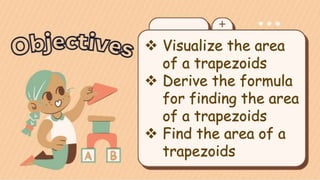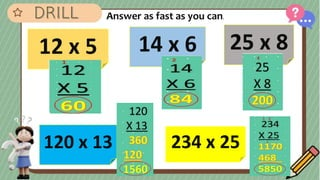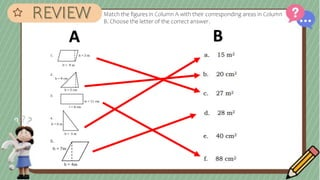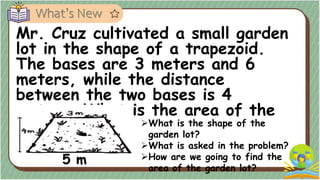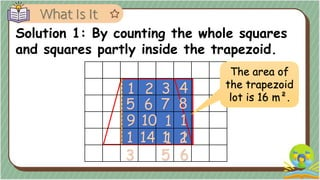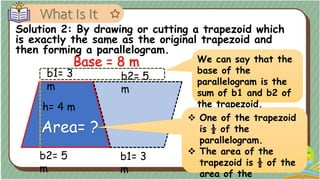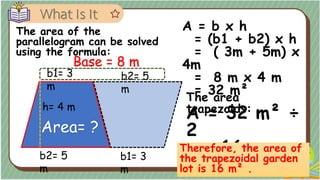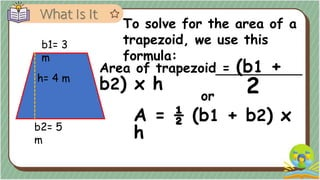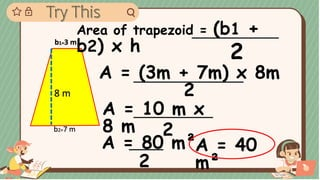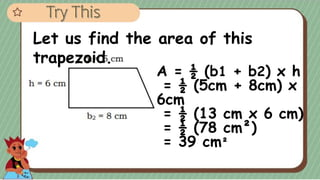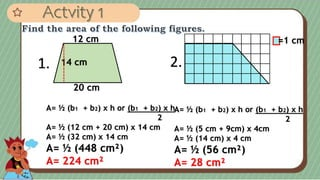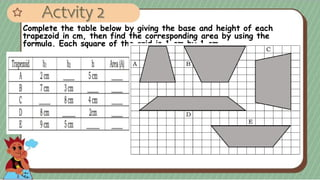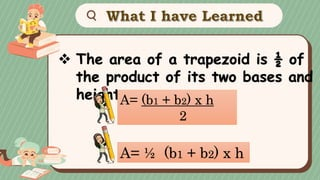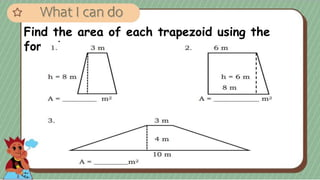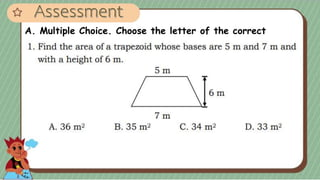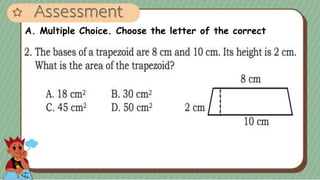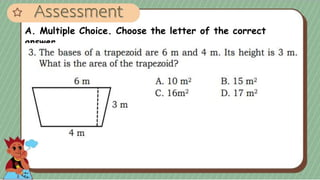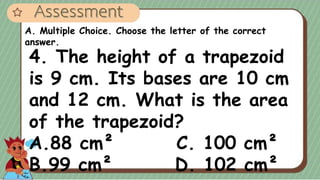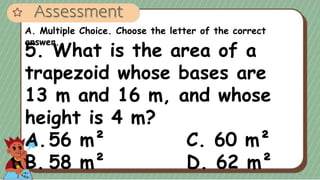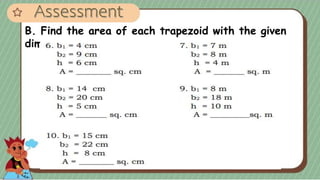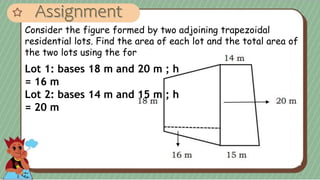1 de 23

### Q4-MATH4-WEEK 2- LESSON 4- FINDING THE AREA OF TRAPEZOIDS.pptx

• 2.  Visualize the area of a trapezoids  Derive the formula for finding the area of a trapezoids  Find the area of a trapezoids
• 3. Answer as fast as you can. 12 x 5 234 x 25 120 x 13 25 x 8 14 x 6
• 4. Match the figures in Column A with their corresponding areas in Column B. Choose the letter of the correct answer. A B
• 5. Mr. Cruz cultivated a small garden lot in the shape of a trapezoid. The bases are 3 meters and 6 meters, while the distance between the two bases is 4 meters. What is the area of the lot? What is the shape of the garden lot? What is asked in the problem? How are we going to find the area of the garden lot? 5 m
• 6. To find the area of the garden lot, we need to find the area of the trapezoid. The lot has an upper base of 3 meters and a lower base of 5 meters. Its height is 4 meters. Base 1= 3 m Base 2 = 5 m Height = 4 m
• 7. Solution 1: By counting the whole squares and squares partly inside the trapezoid. 1 2 3 4 5 6 7 8 9 10 1 1 1 2 1 3 14 1 5 1 6 The area of the trapezoid lot is 16 m².
• 8. Solution 2: By drawing or cutting a trapezoid which is exactly the same as the original trapezoid and then forming a parallelogram. b1= 3 m b2= 5 m h= 4 m b1= 3 m b2= 5 m We can say that the base of the parallelogram is the sum of b1 and b2 of the trapezoid. Area= ?  One of the trapezoid is ½ of the parallelogram.  The area of the trapezoid is ½ of the area of the Base = 8 m
• 9. The area of the parallelogram can be solved using the formula: b1= 3 m b2= 5 m h= 4 m b1= 3 m b2= 5 m Area= ? Base = 8 m A = b x h = (b1 + b2) x h = ( 3m + 5m) x 4m = 8 m x 4 m = 32 m² The area trapezoids: A = 32 m² ÷ 2 = 16 m Therefore, the area of the trapezoidal garden lot is 16 m² .
• 10. b1= 3 m b2= 5 m h= 4 m Area of trapezoid = (b1 + b2) x h To solve for the area of a trapezoid, we use this formula: 2 or A = ½ (b1 + b2) x h
• 11. 8 m b2=7 m b1=3 m Area of trapezoid = (b1 + b2) x h 2 A = (3m + 7m) x 8m 2 A = 10 m x 8 m 2 A = 80 m² 2 A = 40 m²
• 12. Let us find the area of this trapezoid. A = ½ (b1 + b2) x h = ½ (5cm + 8cm) x 6cm = ½ (13 cm x 6 cm) = ½ (78 cm²) = 39 cm²
• 13. 1. 12 cm 14 cm 20 cm =1 cm A= ½ (b1 + b2) x h or (b1 + b2) x h 2 A= ½ (12 cm + 20 cm) x 14 cm A= ½ (32 cm) x 14 cm A= ½ (448 cm²) A= 224 cm² A= ½ (b1 + b2) x h or (b1 + b2) x h 2 A= ½ (5 cm + 9cm) x 4cm A= ½ (14 cm) x 4 cm A= ½ (56 cm²) A= 28 cm² 2.
• 14. Complete the table below by giving the base and height of each trapezoid in cm, then find the corresponding area by using the formula. Each square of the grid is 1 cm by 1 cm.
• 15.  The area of a trapezoid is ½ of the product of its two bases and height. A= (b1 + b2) x h 2 A= ½ (b1 + b2) x h
• 16. Find the area of each trapezoid using the formula.
• 17. A. Multiple Choice. Choose the letter of the correct answer.
• 18. A. Multiple Choice. Choose the letter of the correct answer.
• 19. A. Multiple Choice. Choose the letter of the correct answer.
• 20. A. Multiple Choice. Choose the letter of the correct answer. 4. The height of a trapezoid is 9 cm. Its bases are 10 cm and 12 cm. What is the area of the trapezoid? A.88 cm² C. 100 cm² B.99 cm² D. 102 cm²
• 21. A. Multiple Choice. Choose the letter of the correct answer. 5. What is the area of a trapezoid whose bases are 13 m and 16 m, and whose height is 4 m? A.56 m² C. 60 m² B. 58 m² D. 62 m²
• 22. B. Find the area of each trapezoid with the given dimensions.
• 23. Consider the figure formed by two adjoining trapezoidal residential lots. Find the area of each lot and the total area of the two lots using the formula. Lot 1: bases 18 m and 20 m ; h = 16 m Lot 2: bases 14 m and 15 m ; h = 20 m